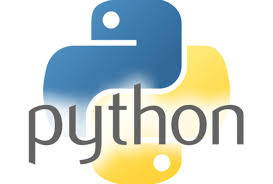41.How will you convert a string to a set in python?
set(s) − Converts s to a set.

42.How will you create a dictionary using tuples in python?
dict(d) − Creates a dictionary. d must be a sequence of (key,value) tuples.

43.How will you convert a string to a frozen set in python?
frozenset(s) − Converts s to a frozen set.

44.How will you convert an integer to a character in python?
chr(x) − Converts an integer to a character.

45.How will you convert an integer to an unicode character in python?
unichr(x) − Converts an integer to a Unicode character.

46.How will you convert a single character to its integer value in python?
ord(x) − Converts a single character to its integer value.

47.How will you convert an integer to hexadecimal string in python?
hex(x) − Converts an integer to a hexadecimal string.

48.How will you convert an integer to octal string in python?
oct(x) − Converts an integer to an octal string.

49.What is the purpose of ** operator?
** Exponent − Performs exponential (power) calculation on operators. a**b = 10 to the power 20 if a = 10 and b = 20.

50.What is the purpose of // operator?
// Floor Division − The division of operands where the result is the quotient in which the digits after the decimal point are removed.